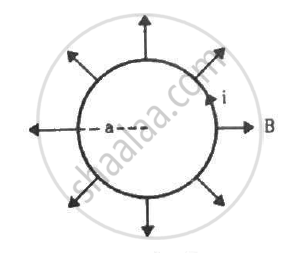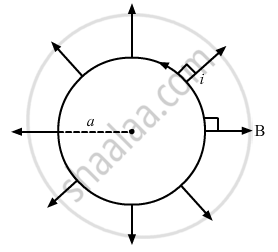Department of Pre-University Education, KarnatakaPUC Karnataka Science Class 12
Advertisement Remove all ads

# A Circular Loop of Radius A, Carrying a Current I, is Placed in a Two-dimensional Magnetic Field. the Centre - Physics

Sum

A circular loop of radius a, carrying a current i, is placed in a two-dimensional magnetic field. The centre of the loop coincides with the centre of the field (figure). The strength of the magnetic field at the periphery of the loop is B. Find the magnetic force on the wire.Advertisement Remove all ads

#### Solution

Given:-

A circular loop of radius = a

So, the length of the loop, l = 2πa

Electric current through the loop = i

As per the question,

The loop is placed in a two-dimensional magnetic field. The centre of the loop coincides with the centre of the field. The strength of the magnetic field at the periphery of the loop is B

Therefore, the magnetic field points radially outwards.

Here, the angle between the length of the loop and the magnetic field, θ = 90˚

Magnetic force is given by

|vecF| = iveclxx vecB

vecF = i(2piaxxvecB)

vecB = i2piaBDirection of the force can be found using Fleming's left-hand rule.

Thus, the direction of magnetic force is perpendicular to the plane of the figure and pointing inside.

Concept: Torque on a Current Loop in Magnetic Field
Is there an error in this question or solution?
Advertisement Remove all ads

#### APPEARS IN

HC Verma Class 11, Class 12 Concepts of Physics Vol. 2
Chapter 12 Magnetic Field
Q 12 | Page 231
Advertisement Remove all ads

#### Video TutorialsVIEW ALL 

Advertisement Remove all ads
Share
Notifications

View all notifications

Forgot password?